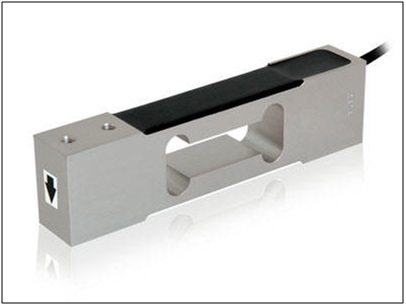LOAD CELL is an electromechanical sensor which functions to measure the amount of static and dynamic forces acting on it Load cell consists of an elastic material that will deform according to the force it receives, the magnitude of this deformation is proportional to the magnitude of force.

To measure the magnitude of the deformation of the deformation of the material, one of the popular ways is to use the “strain gauge

Some types of load cells can be expressed here:

Strain gauges e1 and e3 are installed in opposite directions and measure axial strains. The e2 and e4 gauge strains are installed face to face and measure circumferential strains. The value of each strain can be described as follows:

e1 = e3 = t/E = F/AE
ePV2PV =e4 = – v F/AE